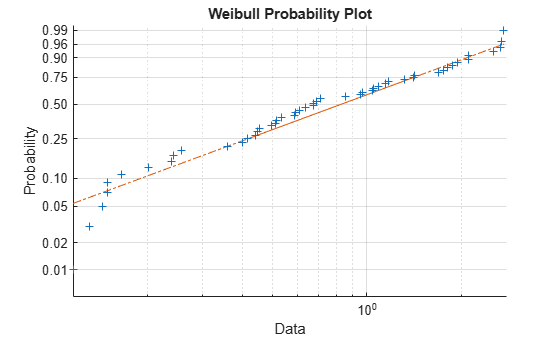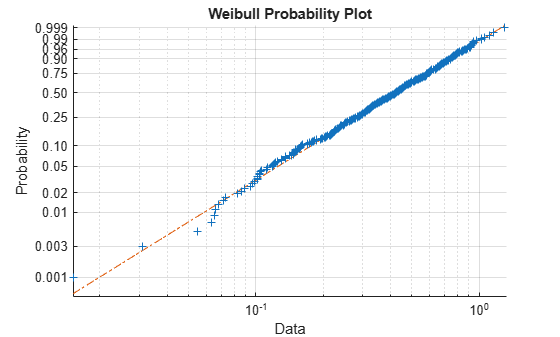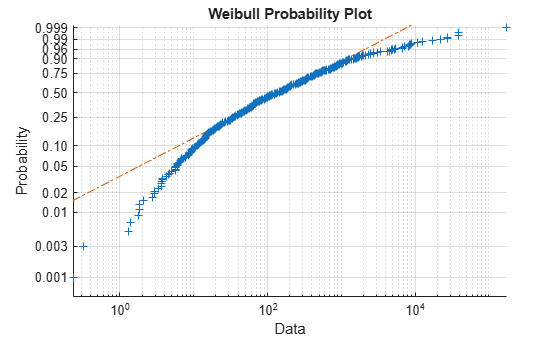# wblplot

Weibull probability plot

## Syntax

``wblplot(x)``
``wblplot(ax,x)``
``h = wblplot(___)``

## Description

example

````wblplot(x)` creates a Weibull probability plot comparing the distribution of the data in `x` to the Weibull distribution.`wblplot` plots each data point in `x` using plus sign (`'+'`) markers and draws two reference lines that represent the theoretical distribution. A solid reference line connects the first and third quartiles of the data, and a dashed reference line extends the solid line to the ends of the data. If the sample data has a Weibull distribution, then the data points appear along the reference line. A distribution other than Weibull introduces curvature in the data plot.```
````wblplot(ax,x)` adds a Weibull probability plot into the axes specified by `ax`.```
````h = wblplot(___)` returns graphics handles corresponding to the plotted lines, using any of the input arguments in the previous syntaxes.```

## Examples

collapse all

Generate a vector `r` containing 50 random numbers from the Weibull distribution with the scale parameter 1.2 and the shape parameter 1.5.

```rng('default') % For reproducibility r = wblrnd(1.2,1.5,50,1);```

Create a Weibull probability plot to visually determine if the data comes from a Weibull distribution.

`wblplot(r)`The plot indicates that the data likely comes from a Weibull distribution.

Generate two sample data sets, one from a Weibull distribution and another from a lognormal distribution. Perform the Lilliefors test to assess whether each data set is from a Weibull distribution. Confirm the test decision by performing a visual comparison using a Weibull probability plot (`wblplot`).

Generate samples from a Weibull distribution.

```rng('default') data1 = wblrnd(0.5,2,[500,1]);```

Perform the Lilliefors test by using the `lillietest`. To test data for a Weibull distribution, test if the logarithm of the data has an extreme value distribution.

`h1 = lillietest(log(data1),'Distribution','extreme value')`
```h1 = 0 ```

The returned value of `h1 = 0` indicates that `lillietest` fails to reject the null hypothesis at the default 5% significance level. Confirm the test decision using a Weibull probability plot.

`wblplot(data1)`The plot indicates that the data follows a Weibull distribution.

Generate samples from a lognormal distribution.

`data2 =lognrnd(5,2,[500,1]);`

Perform the Lilliefors test.

`h2 = lillietest(log(data2),'Distribution','extreme value')`
```h2 = 1 ```

The returned value of `h2 = 1` indicates that `lillietest` rejects the null hypothesis at the default 5% significance level. Confirm the test decision using a Weibull probability plot.

`wblplot(data2)`The plot indicates that the data does not follow a Weibull distribution.

## Input Arguments

collapse all

Sample data, specified as a numeric vector or numeric matrix. `wblplot` displays each value in `x` using the symbol `'+'`. If `x` is a matrix, then `wblplot` displays a separate line for each column of `x`.

Data Types: `single` | `double`

Target axes, specified as an `Axes` object or a `UIAxes` object. `wblplot` adds an additional plot into the axes specified by `ax`. For details, see Axes Properties and UIAxes Properties.

Use `gca` to return the current axes for the current figure.

## Output Arguments

collapse all

Graphics handles for line objects, returned as a vector of `Line` graphics handles. Graphics handles are unique identifiers that you can use to query and modify the properties of a specific line on the plot. For each column of `x`, `wblplot` returns three handles:

• The line representing the data points. `wblplot` represents each data point in `x` using plus sign (`'+'`) markers.

• The line joining the first and third quartiles of each column of `x`, represented as a solid line.

• The extrapolation of the quartile line, extended to the minimum and maximum values of `x`, represented as a dashed line.

To view and set properties of line objects, use dot notation. For information on using dot notation, see Access Property Values. For information on the `Line` properties that you can set, see Line Properties.

## Algorithms

`wblplot` matches the quantiles of sample data to the quantiles of a Weibull distribution. The sample data is sorted, scaled logarithmically, and plotted on the x-axis. The y-axis represents the quantiles of the Weibull distribution, converted into probability values. Therefore, the y-axis scaling is not linear.

Where the x-axis value is the ith sorted value from a sample of size N, the y-axis value is the midpoint between evaluation points of the empirical cumulative distribution function of the data. The midpoint is equal to $\frac{\left(i-0.5\right)}{N}$.

`wblplot` superimposes a reference line to assess the linearity of the plot. The line goes through the first and third quartiles of the data.

## Alternative Functionality

You can use the `probplot` function to create a probability plot. The `probplot` function enables you to indicate censored data and specify the distribution for a probability plot.

## Version History

Introduced before R2006a